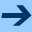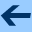Program Control

# Program Control

GOO provides a variety of program control constructs including function calls, conditional execution, and nonlocal control flow.
 SEQ (SEQ ,@forms) S evaluates forms sequentially and returns values of evaluating last form (cf. Scheme's BEGIN) (SEQ) S returns false IF (IF ,test ,then [ ,else ]) S evaluates either ,then if ,test is non-false otherwise evaluates ,else (cf. Scheme's IF). The ,else expression defaults to false. AND (AND ,form ,@forms) S == (IF ,form (AND ,@FORMS)) (AND ,form) S == ,form OR (OR ,form ,@forms) S == (SEQ (DEF x ,form) (IF x x (OR ,@FORMS))) (OR ,form) S == ,form UNLESS (UNLESS ,test ,@body) S == (IF (NOT ,test) (SEQ ,@body)) WHEN (WHEN ,test ,@body) S == (IF ,test (SEQ ,@body)) COND (COND (,test ,@body) ...) S evaluates (SEQ ,@body) of first clause whose ,test evaluates to non-false (cf. Dylan's CASE and Scheme's COND). CASE[-BY] (CASE[-BY] ,value [ ,test ]((,@keys) ,@body) ...) S evaluates ,value and then evaluates (SEQ ,@body) of first clause for which (,test ,value ,key) returns non-false (cf. Dylan's SELECT and Scheme's CASE). N.B., each key is evaluated, thus symbols must be quoted. The default ,test for the CASE form is ==. OPF (OPF ,place ,expr) S == (SEQ (DEF _ ,place) (SET ,place ,expr)), where ,place is evaluated only once. For example, (OPF x (+ _ 1)) == (SET x (+ x 1)). SWAPF (SWAPF ,x ,y) S == (SEQ (DEF tmp ,x) (SET ,x ,y) (SET ,y tmp)), where ,x and ,y are evaluated only once. ROTF (ROTF ,x ,y ,@places) S == (SET (TUP ,x ,y ,@places) (TUP ,y ,@places ,x)) where ,x, ,y, and ,@places) are evaluated only once and (SET (TUP ...) val) stands for parallel assignment. call (,f ,@args) S evaluates ,f and then ,@args in left to right order and then calls ,f with the evaluated arguments. REP (REP ,name ((,var ,init) ...) ,@body) S defines a recursive loop (cf., Dylan's ITERATE or Scheme's (LET ,var ...)). ESC (ESC ,name ,@body) S evaluates (SEQ ,@body) with an exit function of a single parameter, x, bound to ,name that if called, will cause ESC to return the value of x (cf. Dylan's BLOCK/RETURN). It is illegal to call the exit function after the execution of the creating ESC form (i.e., no upward continuations). FIN (FIN ,protected ,@cleanups) S ensures that (SEQ ,@cleanups) is evaluated whether or not an ESC upwards exit is taken during the dynamic-extent of ,protected (cf. Dylan's BLOCK/CLEANUP form and CL's UNWIND-PROTECT). The result of a FIN form is the result of evaluating its protected form. ASSERT (ASSERT ,test ,message ,@args) S == (UNLESS ,test (ERROR ,message ,@args))Program Control